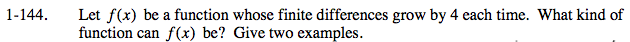### Home > CALC > Chapter 1 > Lesson 1.4.1 > Problem1-144

1-144.Finite differences can be used to analyze the slope of a graph at various x-values. In Lesson 1.3.1, you found consistent patterns in the way polynomial functions change. Which polynomial function would have finite differences that grow by 4 each time?

f(x) is quadratic, for example, f(x) = 2x2.